## Implement Box-Jenkins Model Selection and Estimation Using Econometric Modeler App

This example shows how to use the Box-Jenkins methodology to select and estimate an ARIMA model by using the Econometric Modeler app. Then, it shows how to export the estimated model to generate forecasts. The data set, which is stored in `Data_JAustralian.mat`, contains the log quarterly Australian Consumer Price Index (CPI) measured from 1972 and 1991, among other time series.

### Prepare Data for Econometric Modeler

At the command line, load the `Data_JAustralian.mat` data set.

`load Data_JAustralian`

Convert the table `DataTable` to a timetable:

1. Clear the row names of `DataTable`.

2. Convert the sampling times to a `datetime` vector.

3. Convert the table to a timetable by associating the rows with the sampling times in `dates`.

```DataTable.Properties.RowNames = {}; dates = datetime(dates,'ConvertFrom','datenum',... 'Format','ddMMMyyyy','Locale','en_US'); DataTable = table2timetable(DataTable,'RowTimes',dates);```

### Import Data into Econometric Modeler

At the command line, open the Econometric Modeler app.

`econometricModeler`

Alternatively, open the app from the apps gallery (see Econometric Modeler).

Import `DataTable` into the app:

1. On the Econometric Modeler tab, in the Import section, click.

2. In the Import Data dialog box, in the Import? column, select the check box for the `DataTable` variable.

3. Click .

The variables, including `PAU`, appear in the Data Browser, and a time series plot of all the series appears in the Time Series Plot(EXCH) figure window.

Create a time series plot of `PAU` by double-clicking `PAU` in the Data Browser.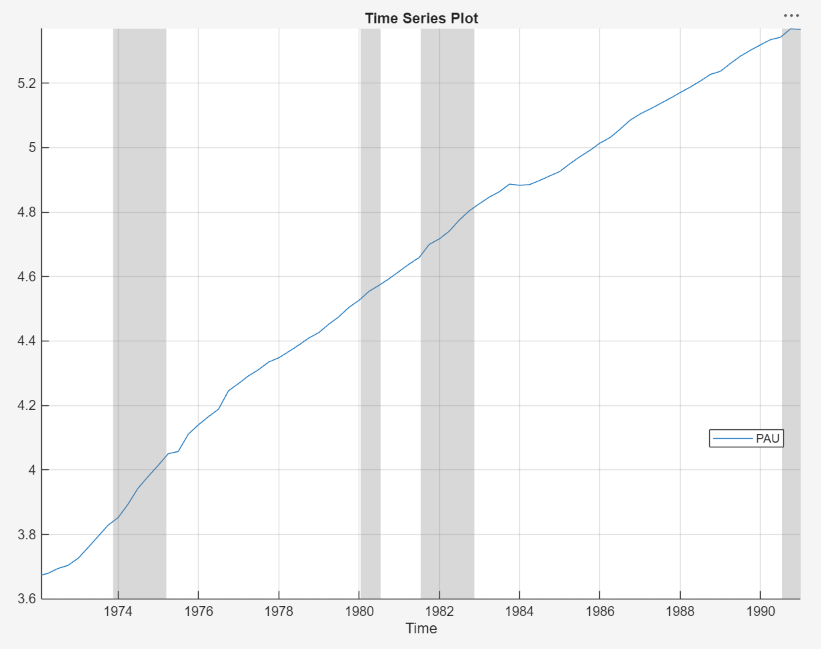The series appears nonstationary because it has a clear upward trend.

### Plot Sample ACF and PACF of Series

Plot the sample autocorrelation function (ACF) and partial autocorrelation function (PACF).

1. In the Data Browser, select the `PAU` time series.

2. Click the Plots tab, then click .

3. Click the Plots tab, then click .

4. Close all figure windows except for the correlograms. Then, drag the ACF(PAU) figure window above the PACF(PAU) figure window.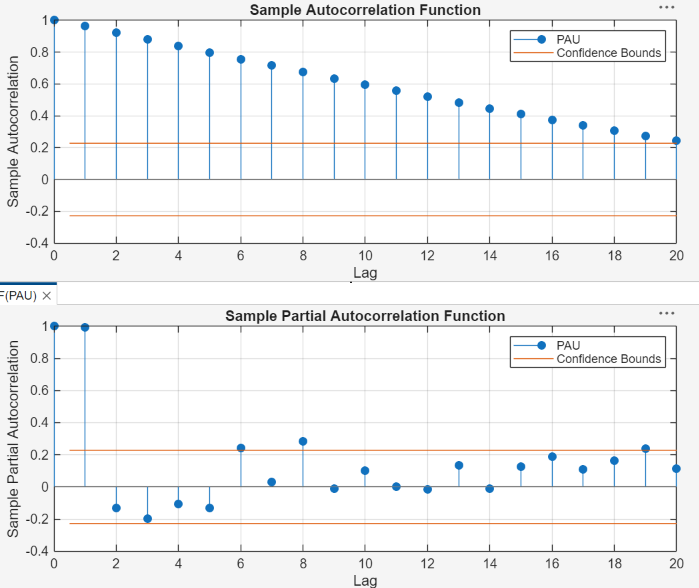The significant, linearly decaying sample ACF indicates a nonstationary process.

Close the ACF(PAU) and PACF(PAU) figure windows.

### Difference the Series

Take a first difference of the data. With `PAU` selected in the Data Browser, on the Econometric Modeler tab, in the Transforms section, click .

The transformed variable `PAUDiff` appears in the Data Browser, and its time series plot appears in the Time Series Plot(PAUDiff) figure window.Differencing removes the linear trend. The differenced series appears more stationary.

### Plot Sample ACF and PACF of Differenced Series

Plot the sample ACF and PACF of `PAUDiff`. With `PAUDiff` selected in the Data Browser:

1. Click the Plots tab, then click .

2. Click the Plots tab, then click .

3. Close the Time Series Plot(PAUDiff) figure window. Then, drag the ACF(PAUDiff) figure window above the PACF(PAUDiff) figure window.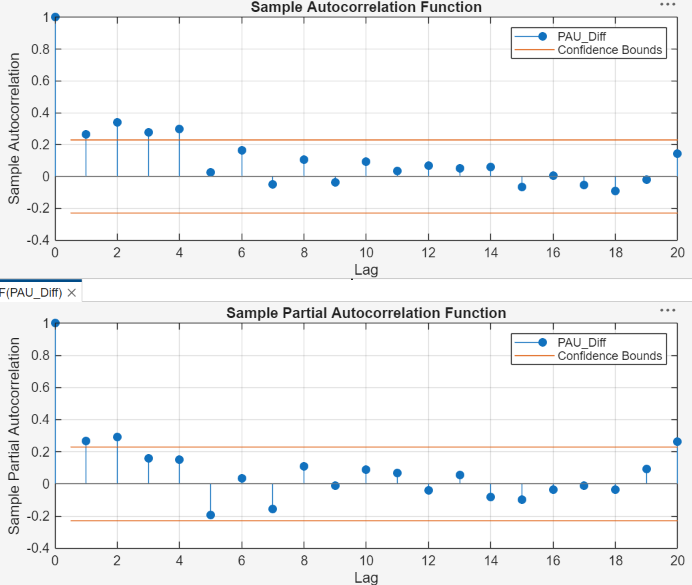The sample ACF of the differenced series decays more quickly. The sample PACF cuts off after lag 2. This behavior is consistent with a second-degree autoregressive (AR(2)) model for the differenced series.

Close the ACF(PAUDiff) and PACF(PAUDiff) figure windows.

### Specify and Estimate ARIMA Model

Estimate an ARIMA(2,1,0) model for the log quarterly Australian CPI. This model has one degree of nonseasonal differencing and two AR lags.

1. In the Data Browser, select the `PAU` time series.

2. On the Econometric Modeler tab, in the Models section, click ARIMA.

3. In the ARIMA Model Parameters dialog box, on the Lag Order tab:

1. Set Degree of Integration to `1`.

2. Set Autoregressive Order to `2`.4. Click .

The model variable `ARIMA_PAU` appears in the Models section of the Data Browser, and its estimation summary appears in the Model Summary(ARIMA_PAU) document.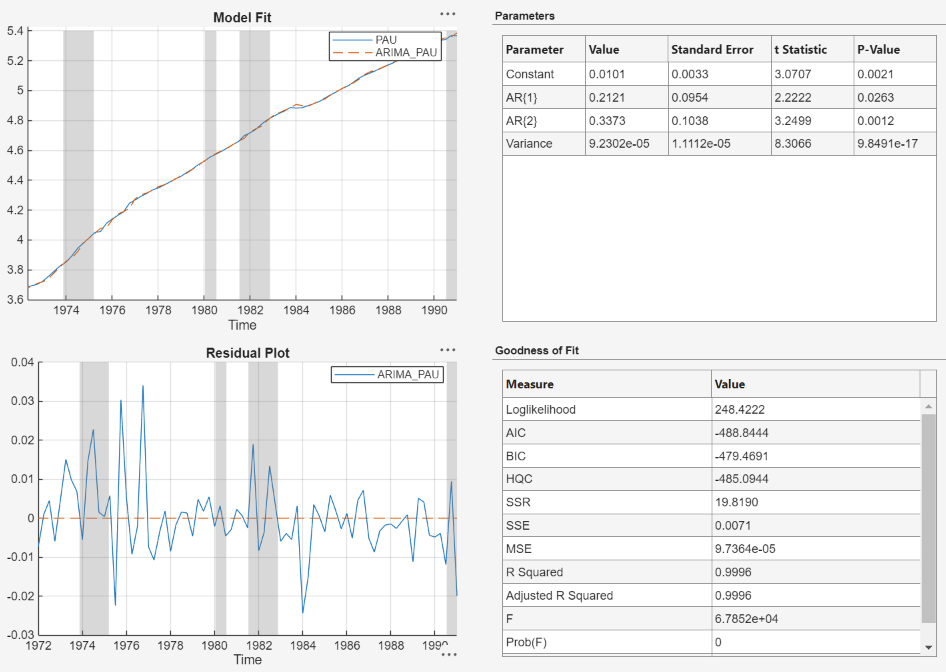Both AR coefficients are significant at a 5% significance level.

### Check Goodness of Fit

Check that the residuals are normally distributed and uncorrelated by plotting a histogram, quantile-quantile plot, and ACF of the residuals.

1. Close the Model Summary(ARIMA_PAU) document.

2. With `ARIMA_PAU` selected in the Data Browser, on the Econometric Modeler tab, in the Diagnostics section, click > Residual Histogram.

3. Click > Residual Q-Q Plot.

4. Click > Autocorrelation Function.

5. In the right pane, drag the Histogram(ARIMA_PAU) and QQPlot(ARIMA_PAU) figure windows so that they occupy the upper two quadrants, and drag the ACF so that it occupies the lower two quadrants.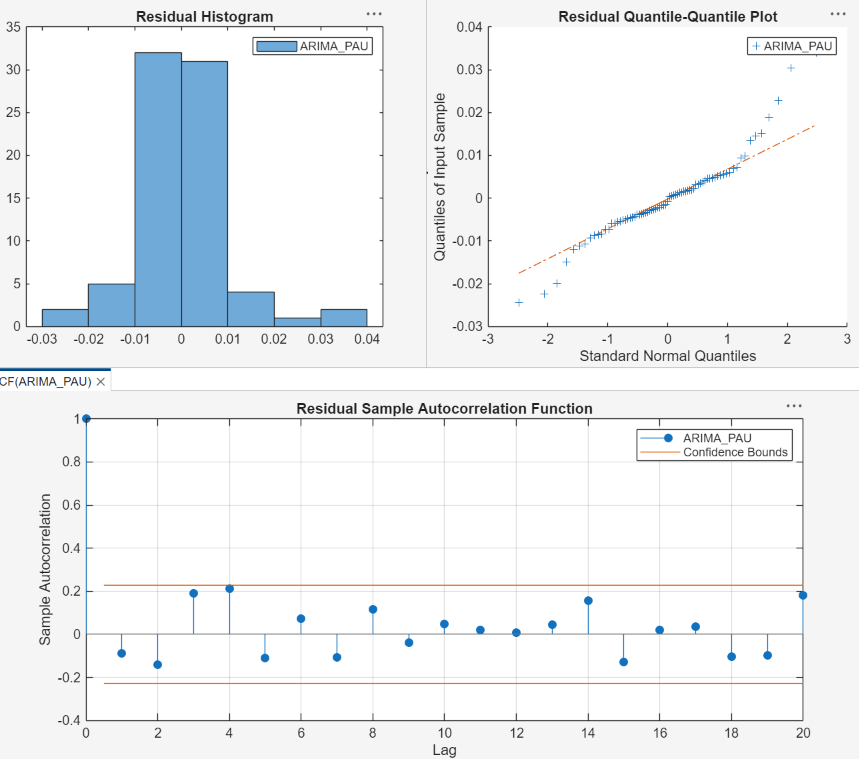The residual plots suggest that the residuals are approximately normally distributed and uncorrelated. However, there is some indication of an excess of large residuals. This behavior suggests that a t innovation distribution might be appropriate.

### Export Model to Workspace

Export the model to the MATLAB® Workspace.

1. In the Data Browser, select the `PAU` time series.

2. On the Econometric Modeler tab, in the Export section, click > .

3. In the Export Variables dialog box, select the Select check box for the ARIMA_PAU model.

4. Click . The check box for the PAU time series is already selected.

The variables `PAU` and `ARIMA_PAU` appear in the workspace.

### Generate Forecasts at Command Line

Generate forecasts and approximate 95% forecast intervals from the estimated ARIMA(2,1,0) model for the next four years (16 quarters). Use the entire series as a presample for the forecasts.

```[PAUF,PAUMSE] = forecast(ARIMA_PAU,16,'Y0',PAU); UB = PAUF + 1.96*sqrt(PAUMSE); LB = PAUF - 1.96*sqrt(PAUMSE); datesF = dates(end) + calquarters(1:16); figure h4 = plot(dates,PAU,'Color',[.75,.75,.75]); hold on h5 = plot(datesF,PAUF,'r','LineWidth',2); h6 = plot(datesF,UB,'k--','LineWidth',1.5); plot(datesF,LB,'k--','LineWidth',1.5); legend([h4,h5,h6],'Log CPI','Forecast',... 'Forecast Interval','Location','Northwest') title('Log Australian CPI Forecast') hold off```Box, G. E. P., G. M. Jenkins, and G. C. Reinsel. Time Series Analysis: Forecasting and Control. 3rd ed. Englewood Cliffs, NJ: Prentice Hall, 1994.